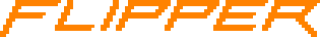# [HELP] Signal analysing from Flipper to Universal Radio Hacker

Hi!
I want to analyse signals I captured with my Flipper on Universal Radio Hacker (https://github.com/jopohl/urh).

The problem is that URH can’t open Flipper’s .sub files. I’m fluent in python, so I’m willing to write a script to convert the .sub to something that URH will understand.

The problem is that I’m not sure what URH wants. I can convert the data to CSV but URH is trunking it at 100 lines. I think WAV files will work, but I’m not sure how the format works. I need help!

2 Likes

cool approach!

it is possible to read large csv files into URH.
would be nice if you could create a script.

WAV files are definitely not the way to go.

I managed to massage a .sub file to CSV and read it into PulseView - I was planning to do the analysis there but it doesn’t look like it is decoding properly. Still have to try to read that into URH.

BTW, here’s the code I used to convert from .sub to .csv (python3):
import sys

``````CSV         = True      # write to CSV
MAX_SAMPLES = 5000000   # max number of samples to write to file
CONT_DATA   = True      # interpolate original data?
SAMPLE_SIZE = 1         # divide the timestamp data by this value
DECIMATION  = 10       # only use every N samples

f = open(sys.argv)

data = a.split('\n')
samples = []
for d in data:
if "RAW_Data" in d:
samples += (d.split(': ').split(' '))

print("Samples on .sub file: ", len(samples))

values = []
t = 0

for s in samples:
bit_val = 1 if int(s) >= 0 else 0
if CONT_DATA == False:
t = t + abs(int(s))/SAMPLE_SIZE
values.append((t, bit_val))
else:
for u in range(abs(int(s))):
t += 1/SAMPLE_SIZE
values.append((t, bit_val))

# decimation
dec_val = []
i = 0
for v in values:
i += 1
if i % DECIMATION == 0:
dec_val.append(v)
values = dec_val

if CSV is True:
f = open("converted_sig.csv", "w")
f.write("Time[s], Ch2 \n")
samples = 0
print("Samples on CSV file:", min(len(values), MAX_SAMPLES))
for v in values:
f.write("{:.16f}".format(v) + ", " + str(v) + "\n")
samples += 1
if samples >= MAX_SAMPLES: break

f.close()

print("Done.")``````